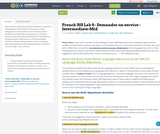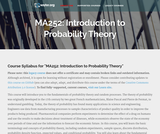# Search Results (3)

View
Selected filters:
• ConditionalConditions of Use:
Remix and Share
Rating

This activity is intended to provide authentic material to Intermediate--Mid students. The purpose of this assignment is to help learners use recently studied vocabulary and idiomatic expressions in a situation similar to one that they would likely find themselves in during authentic experiences in French speaking countries. Studens will be asked to create a dialogue demonstrating their understanding of new vocabulary to ask for help or for a service.

Subject:
Languages
Material Type:
Activity/Lab
Author:
Amber Hoye
Emily Blackburn
Camille Daw
Mimi Fahnstrom
12/11/2019Conditions of Use:
Rating

In this course, the student will learn the basic terminology and concepts of probability theory, including sample size, random experiments, outcome spaces, discrete distribution, probability density function, expected values, and conditional probability. The course also delves into the fundamental properties of several special distributions, including binomial, geometric, normal, exponential, and Poisson distributions. Upon successful completion of this course, the student will be able to: Define probability, outcome space, events, and probability functions; Use combinations to evaluate the probability of outcomes in coin-flipping experiments; Calculate the union of events and conditional probability; Apply Bayes's theorem to simple situations; Calculate the expected values of discrete and continuous distributions; Calculate the sums of random variables; Calculate cumulative distributions and marginal distributions; Evaluate random processes governed by binomial, multinomial, geometric, exponential, normal, and Poisson distributions; Define the law of large numbers and the central limit theorem. (Mathematics 252)

Subject:
Statistics and Probability
Material Type:
Full Course
Provider:
The Saylor Foundation
11/11/2011Conditions of Use:
No Strings Attached
Rating

This module defines several key terms related to Probability. The original module by Susan Dean and Dr. Barbara Illowsky in the textbook collection Collaborative Statistics has been modified by Roberta Bloom.

Subject:
Statistics and Probability
Material Type: